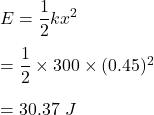## How much elastic energy is stored in a slingshot that has a spring constant of 300 N/m and is stretched by 0.45m?

Question

How much elastic energy is stored in a slingshot that has a spring constant of 300 N/m and is stretched by 0.45m?

in progress 0
2 months 2021-07-25T02:39:18+00:00 1 Answers 1 views 0

E = 30.37 J

Step-by-step explanation:

Given that,

The spring constant of the spring, k = 300 N/m

The spring is stretched by 0.45 m, x = 0.45 m

We need to find the elastic energy stored in the spring. The formula for the elastic energy is given by :So, the required elastic energy is equal to 30.37 J.